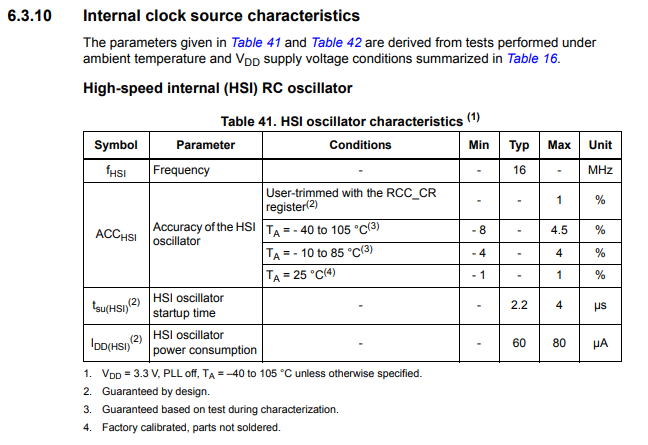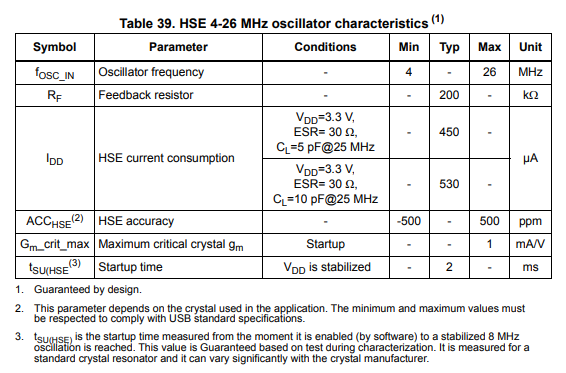﻿ HSI vs HSE Clock in an STM32F4xx Microcontroller Board- Explained ﻿# HSI vs HSE Clock in an STM32F4xx Microcontroller Board- ExplainedIn this article, we explain the differences between an HSI and and an HSE clock in an STM32F4xx microcontroller board.

The HSI clock is the internal RC oscillator clock that comes with the microcontroller board.

The HSI clock consists of an RC circuit, which is a resistor-capacitor circuit that oscillates at a specific frequency and can function as the clock.

The HSE clock is the external clock that sometimes comes attached to a microcontroller. Other times, the external circuitry may need to be added.

The HSE normally consists of an external crystal oscillator.

The external crystal oscillator is manufactured to operate at a specific frequency and and, like the HSI, can function as the system clock for a microcontroller. The system clock is the main clock for the entire microcontroller.

There are advantages and disadvantages to using HSI as the clock vs HSE.

Advantages of using the HSI clock as the system clock is a faster startup time, less power consumption, and no need to add any external circuitry to a board. A disadvantage is a less accurate clock signal, which is a clock signal that can vary a good deal, especially based on the ambient temperature.

An advantage of using the HSE clock as the system clock is a much more accurate clock signal. Disadvantages include a longer startup time and more power consumption.

HSI is the default clock for STM32F4xx microcontrollers, so configuring HSE requires more setup.

So now that we know the basics of HSI vs HSE, let's specifically look at the characteristics for each clock, as given by the datasheet.

In our example, we will look at the STM32F446RE microcontroller board.

Below is a table displaying the characteristics for the HSI clock for this microcontroller board.So you can see that we have in the table shown above various characteristics of the clock, including its frequency, accuracy, startup time, and power consumption.

We can see based on this table for this microcontroller, the HSI clock has a frequency of 16MHz.

The accuracy of this HSI clock signal varies according to the ambient temperature. At the standard operating temperature of 25°C, the HSI clock frequency may only vary by -1% to 1%. From a temperature of -10°C to 85°C, the clock frequency may vary by -4 to 4%. From a temperature of -40°C to 105°C, the clock frequency may vary by -8% to 4.5%. Thus, the temperature can make the clock frequency vary by a lot in HSI RC clock systems.

The startup time for this HSI oscillator 2.2μs typically, with a maximum startup time of 4μs.

The power consumption of the HSI oscillator is 60μA typically, with a maximum power consumption of 80μA.

Now let's look at the table displaying the characteristics for the HSE clock for the STM32F446RE microcontroller board.So the external oscillator frequency for the HSE clock can be between 4-26MHz.

The power consumption of the HSE clock is between 450-530μA, which is much more than the power consumption of the HSI clock, which typically used 60μA.

The accuracy of the HSE clock is specified in parts per million (ppm), and we see that this can vary by -500 and 500. So if we use the crystal oscillator frequency of 16MHz to make things equal with the HSI signal, this means that the frequency can vary by ± 8000Hz. This is much more accurate than an HSI signal, which at best can vary by ±1%. 1% of 16MHz would be 160,000Hz, which is considerably more than the 8000Hz which a crystal oscillator may vary by. Under the worse case temperature conditions, in which the temperature may cause an 8% variance in the clock frequency, this can translate into an accuracy loss of 1.28MHz. For an external crystal oscillator, the maximum accuracy loss can only be 8000Hz. Thus, HSE clocks are much more accurate than HSI clock signals.

The startup time for an HSE clock is 2ms typically. This is much longer than the 2.2μs startup time of an HSI clock. Thus, HSI clock signals are more desirable in this category.

Thus, through these comparisons, you can begin to understand the difference between HSI and HSE clock signals.

HSI clock signals use less power and have a quicker startup time than HSE clock signals, but HSE clock signals are much more accurate than HSI clock signals.

The needs of a particular circuit or system can help guide the decision of whether you choose an HSI or an HSE clock signal for a particular microcontroller application.

Related Resources